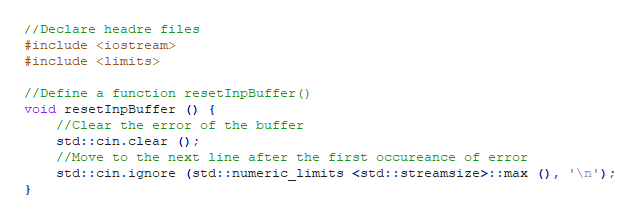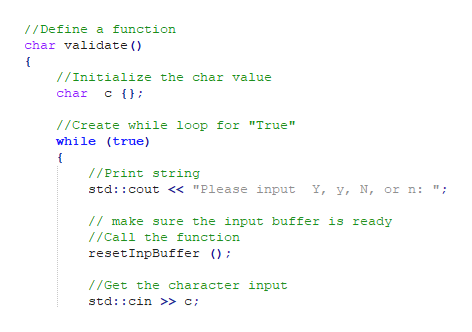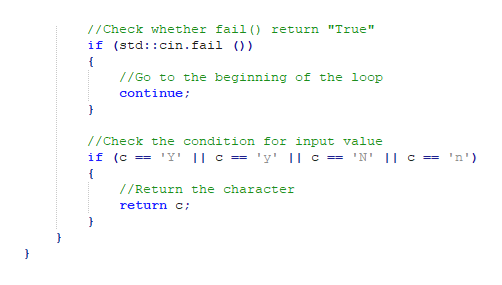# This is my function for validating a char value. So far it doesn't accept any value. I need it to accept Y and N. I can't find the problem. #include <iostream>#include <limits>using std::cin;using std::cout;char validate(char value){ while (std::cin.fail() || (value != 'Y') || (value != 'y') || (value != 'N') || (value != 'n')) {  cout << "Please input a Y or N." << endl;  cin.clear();  cin.ignore(std::numeric_limits<std::streamsize>::max(), '\n');  cin >> value; } return value;}

Question
24 views

This is my function for validating a char value. So far it doesn't accept any value. I need it to accept Y and N. I can't find the problem.

#include <iostream>

#include <limits>

using std::cin;

using std::cout;

char validate(char value)
{
while (std::cin.fail() || (value != 'Y') || (value != 'y') || (value != 'N') || (value != 'n'))
{
cout << "Please input a Y or N." << endl;
cin.clear();
cin.ignore(std::numeric_limits<std::streamsize>::max(), '\n');
cin >> value;
}
return value;
}

check_circle

Step 1

A C++ program for validating a char value is as follows,

#include <iostream>

#include <limits>

//Define a function resetInpBuffer()

void resetInpBuffer () {

//Clear the error of the buffer

std::cin.clear ();

//Move to the next line after the first occureance of error

std::cin.ignore (std::numeric_limits <std::streamsize>::max (), '\n');

}

//Define a function

char validate()

{

//Initialize the char value

char  c {};

//Create while loop for "True"

while (true)

{

//Print string

std::cout << "Please input  Y, y, N, or n: ";

// make sure the input buffer is ready

//Call the function

resetInpBuffer ();

//Get the character input

std::cin >> c;

//Check whether fail() return "True"

if (std::cin.fail ())

{

//Go to the beginning of the loop

continue;

}

//Check the condition for input value

if (c == 'Y' || c == 'y' || c == 'N' || c == 'n')

{

//Return the character

return c;

}

}

}

//Define main() function

int main()

{

//Assign the value return from validate() method

char c = validate();

//Print the value

std::cout<<"The character is "<<c;

//Return "0"

return 0;

}

Screenshot of program #1:help_outlineImage Transcriptionclose//Declare headre files include #include //Define a function resetInpBuffer () void resetInpBuffer { //clear the error of the buffer std: :cin.clear ); /Move to the next line after the first occureance of error std: cin.ignore (std::numeric limits : :max ), \n'); fullscreen
Step 2

Screenshot of program #2:help_outlineImage Transcriptionclose// Define a function char validate ) //Initialize the char value char c //Create while loop for "True" while (true) //Print string std: cout <« "Please input Y, y, N, or n: ". / make sure the input buffer is ready //Call the function resetInpBuffer () //Get the character input std::cin >» c; fullscreen
Step 3

Screenshot of program #3...help_outlineImage Transcriptionclose//Check whether fail () return "True" if (std: :cin.fail )) //Go to the beginning of the loop continue //Check the condition for input value if (c Y' || c == 'y' || c == 'N' || c == 'n') /Return the character return C fullscreen

### Want to see the full answer?

See Solution

#### Want to see this answer and more?

Solutions are written by subject experts who are available 24/7. Questions are typically answered within 1 hour.*

See Solution
*Response times may vary by subject and question.
Tagged in

### Computer Science